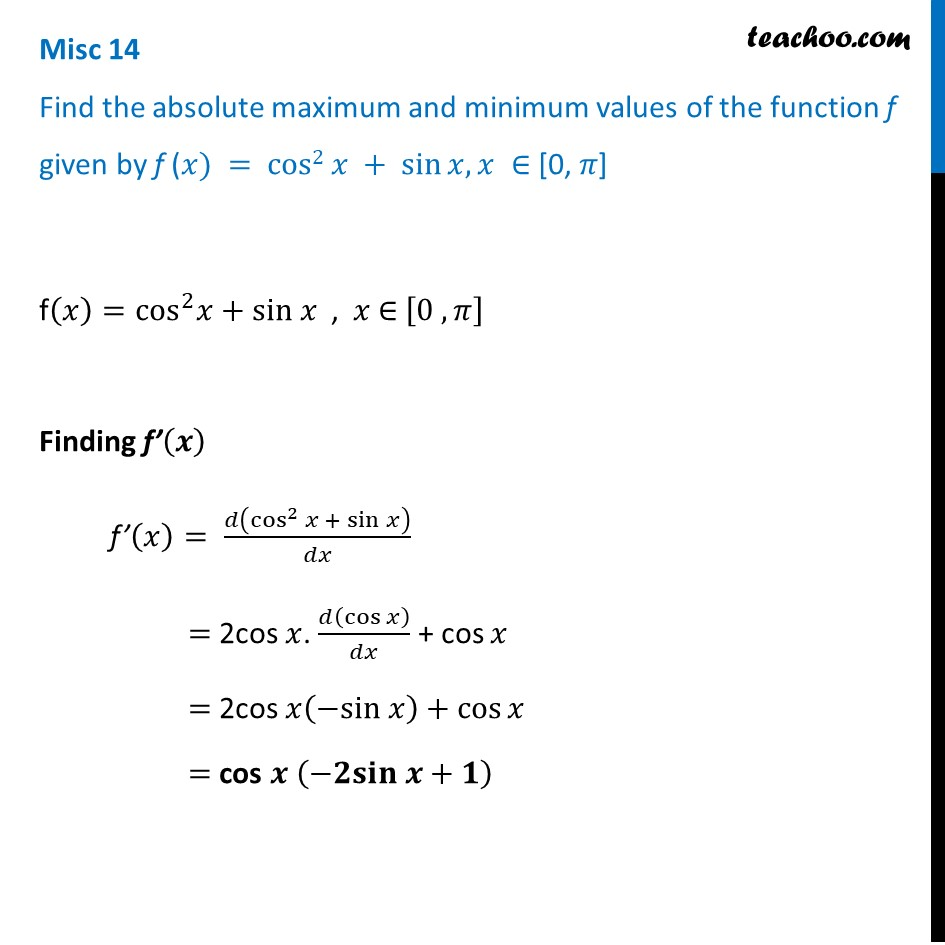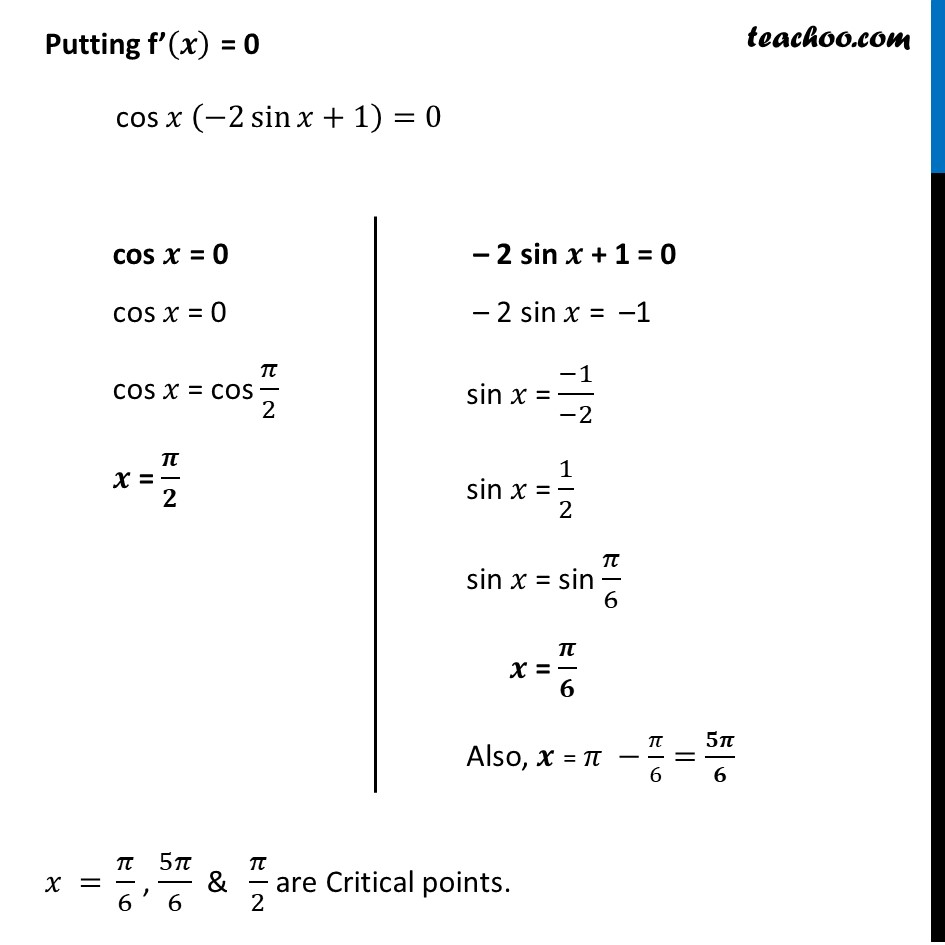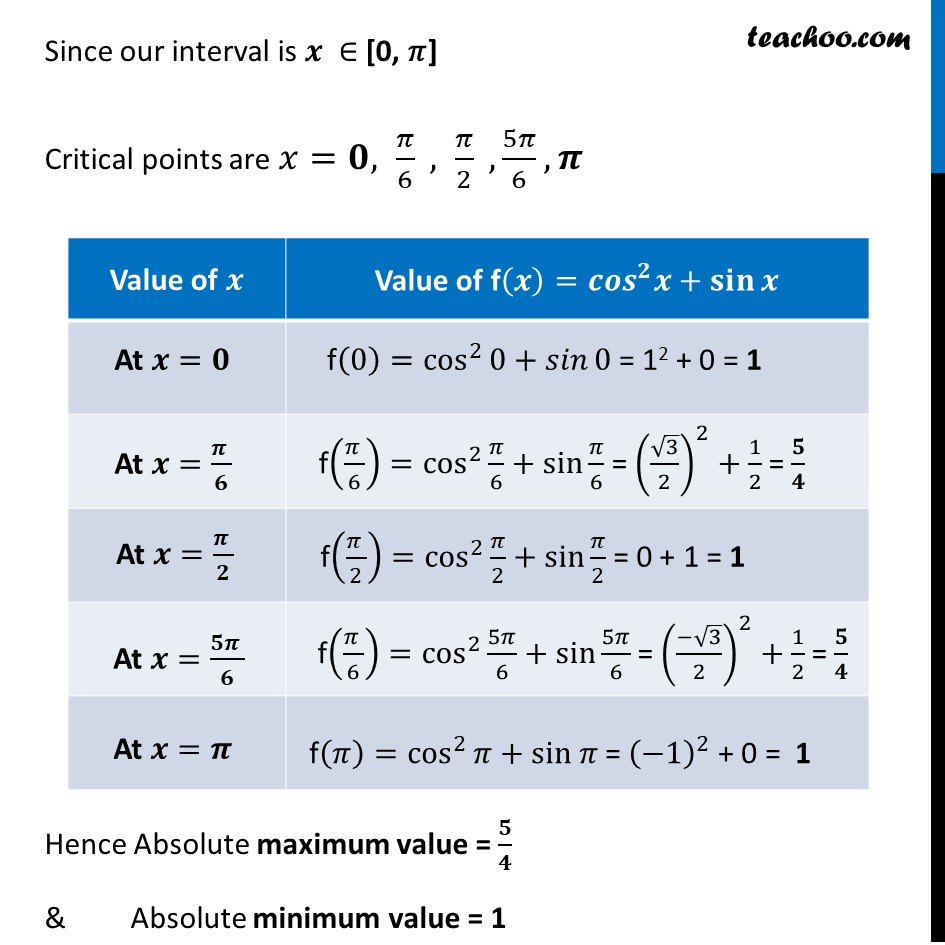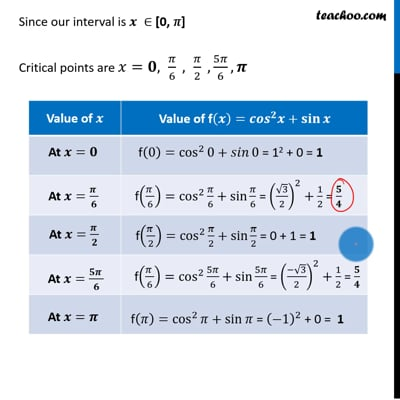Miscellaneous

Chapter 6 Class 12 Application of Derivatives
Serial order wiseThis video is only available for Teachoo black users

Learn in your speed, with individual attention - Teachoo Maths 1-on-1 Class

### Transcript

Misc 11 Find the absolute maximum and minimum values of the function f given by f (𝑥) = cos2 𝑥 + sin⁡𝑥, 𝑥 ∈ [0, 𝜋]f(𝑥)=cos^2 𝑥+sin 𝑥 , 𝑥 ∈ [0 , 𝜋] Finding f’(𝒙) f’(𝑥)= 𝑑(cos^2⁡〖𝑥 + sin⁡𝑥 〗 )/𝑑𝑥 = 2cos 𝑥. 𝑑(cos 𝑥)/𝑑𝑥 + cos 𝑥 = 2cos 𝑥(−sin 𝑥)+cos⁡𝑥 = cos 𝒙 (−𝟐𝐬𝐢𝐧 𝒙+𝟏) Putting f’(𝒙) = 0 cos 𝑥 (−2 sin⁡〖𝑥+1〗 )=0 𝑥 = 𝜋/6 , 5𝜋/6 & 𝜋/2 are Critical points. cos 𝒙 = 0 cos 𝑥 = 0 cos 𝑥 = cos 𝜋/2 𝒙 = 𝝅/𝟐 – 2 sin 𝒙 + 1 = 0 – 2 sin 𝑥 = –1 sin 𝑥 = (−1)/(−2) sin 𝑥 = 1/2 sin 𝑥 = sin 𝜋/6 𝒙 = 𝝅/𝟔 Also, 𝒙 = 𝜋 −𝜋/6=𝟓𝝅/𝟔 Since our interval is 𝒙 ∈ [0, 𝜋] Critical points are 𝑥=𝟎, 𝜋/6 , 𝜋/2 ,5𝜋/6,𝝅 Hence Absolute maximum value = 𝟓/𝟒 & Absolute minimum value = 1